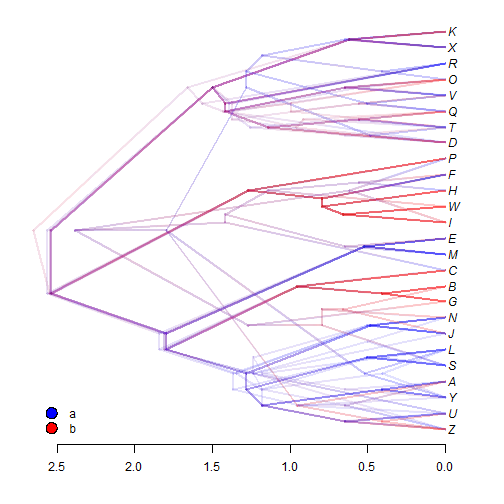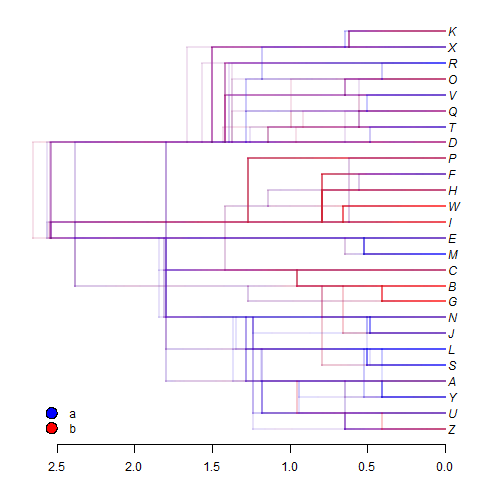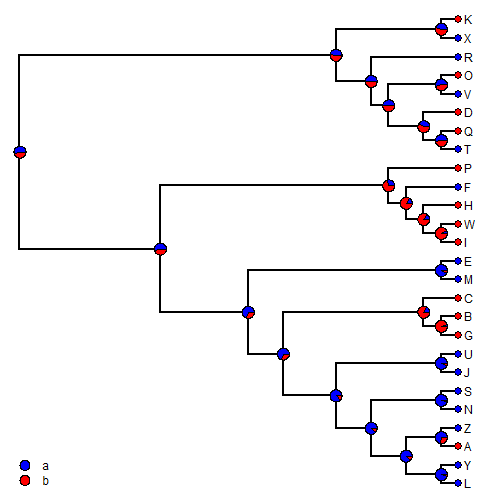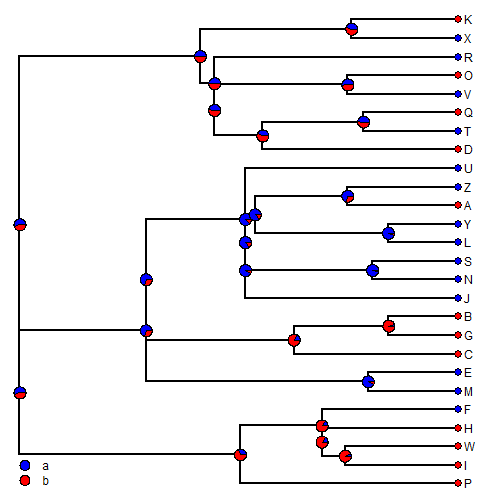## Friday, June 2, 2017

### Summarizing stochastic maps on topologically incongruent trees

Today I received the following inquiry from a phytools user:

“Ideally, I would like to integrate our ASRs over the posterior distribution of trees from BMCMC, but as far as I know, this has not been worked out, and I understand the difficulty given that the node name will be different for each tree. Iâ€™m writing to ask: (1) has there has been any headway on this issue, or is there a workaround beyond simply estimating rates of change? …. The reproducibility and fluidity in graphical representation that R based approaches offer make me reluctant to use other methods….”

The answer is actually that I have implemented a couple of different approaches to summarizing the results from stochastic mapping across trees with incongruent topologies.

1) We can compute a `densityTree` visualization, with the discrete characters mapped.

2) We can compute a consensus topology, and then the posterior probability can be computed as the posterior probability that each node of the consensus tree is in each state, conditioned in the node being present in each of the input trees.

3) Finally, we can input a consensus tree with edge lengths, and compute the posterior probabilities at nodes from our stochastic map trees as the fraction of trees in which each node is present in each state.

Here is a demo using simulated data:

``````## load phytools
library(phytools)
## the data & trees
x
``````
``````## P I W H F M E C G B J U N S L Y A Z D T Q V O R X K
## b b b b a a a b b b a a a a a a b a b a b a b a a b
## Levels: a b
``````
``````trees
``````
``````## 10 phylogenetic trees
``````
``````## compute our stochastic maps
mtrees<-make.simmap(trees,x,nsim=10)
``````
``````## make.simmap is sampling character histories conditioned on the transition matrix
##
## Q =
##            a          b
## a -0.5596168  0.5596168
## b  0.5596168 -0.5596168
## (estimated using likelihood);
## and (mean) root node prior probabilities
## pi =
##   a   b
## 0.5 0.5
``````
``````## Done.
``````
``````## make.simmap is sampling character histories conditioned on the transition matrix
##
## Q =
##           a         b
## a -0.565723  0.565723
## b  0.565723 -0.565723
## (estimated using likelihood);
## and (mean) root node prior probabilities
## pi =
##   a   b
## 0.5 0.5
``````
``````## Done.
``````
``````## make.simmap is sampling character histories conditioned on the transition matrix
##
## Q =
##            a          b
## a -0.4755245  0.4755245
## b  0.4755245 -0.4755245
## (estimated using likelihood);
## and (mean) root node prior probabilities
## pi =
##   a   b
## 0.5 0.5
``````
``````## Done.
``````
``````## make.simmap is sampling character histories conditioned on the transition matrix
##
## Q =
##            a          b
## a -0.5256566  0.5256566
## b  0.5256566 -0.5256566
## (estimated using likelihood);
## and (mean) root node prior probabilities
## pi =
##   a   b
## 0.5 0.5
``````
``````## Done.
``````
``````## make.simmap is sampling character histories conditioned on the transition matrix
##
## Q =
##            a          b
## a -0.5707395  0.5707395
## b  0.5707395 -0.5707395
## (estimated using likelihood);
## and (mean) root node prior probabilities
## pi =
##   a   b
## 0.5 0.5
``````
``````## Done.
``````
``````## make.simmap is sampling character histories conditioned on the transition matrix
##
## Q =
##            a          b
## a -0.5596168  0.5596168
## b  0.5596168 -0.5596168
## (estimated using likelihood);
## and (mean) root node prior probabilities
## pi =
##   a   b
## 0.5 0.5
``````
``````## Done.
``````
``````## make.simmap is sampling character histories conditioned on the transition matrix
##
## Q =
##            a          b
## a -0.5656219  0.5656219
## b  0.5656219 -0.5656219
## (estimated using likelihood);
## and (mean) root node prior probabilities
## pi =
##   a   b
## 0.5 0.5
``````
``````## Done.
``````
``````## make.simmap is sampling character histories conditioned on the transition matrix
##
## Q =
##            a          b
## a -0.5646038  0.5646038
## b  0.5646038 -0.5646038
## (estimated using likelihood);
## and (mean) root node prior probabilities
## pi =
##   a   b
## 0.5 0.5
``````
``````## Done.
``````
``````## make.simmap is sampling character histories conditioned on the transition matrix
##
## Q =
##            a          b
## a -0.5606866  0.5606866
## b  0.5606866 -0.5606866
## (estimated using likelihood);
## and (mean) root node prior probabilities
## pi =
##   a   b
## 0.5 0.5
``````
``````## Done.
``````
``````## make.simmap is sampling character histories conditioned on the transition matrix
##
## Q =
##            a          b
## a -0.5707395  0.5707395
## b  0.5707395 -0.5707395
## (estimated using likelihood);
## and (mean) root node prior probabilities
## pi =
##   a   b
## 0.5 0.5
``````
``````## Done.
``````
``````mtrees
``````
``````## 100 phylogenetic trees with mapped discrete characters
``````

We can start with `densityTree`, as follows:

``````cols=setNames(c("blue","red"),letters[1:2])
method="plotSimmap",colors=cols)
````````````## or I like this style:
densityTree(mtrees,method="plotSimmap",colors=cols)
``````Next, we can let our S3 `summary` method for the object class `"multiSimmap"` compute a consensus tree. It will do this automatically if we set the optional argument `check.equal=TRUE` and it fails:

``````obj<-summary(mtrees,check.equal=TRUE)
``````
``````## Note: Some trees are not equal.
## A "reference" tree will be computed if none was provided.
##
## No reference tree provided & some trees are unequal.
## Computing majority-rule consensus tree.
``````
``````obj
``````
``````## 100 trees with a mapped discrete character with states:
##  a, b
##
## trees have 17.09 changes between states on average
##
## changes are of the following types:
##       a,b  b,a
## x->y 8.71 8.38
##
## mean total time spent in each state is:
##               a          b    total
## raw  15.9786144 14.0835468 30.06216
## prop  0.5315621  0.4684379  1.00000
``````
``````plot(obj,colors=cols)
``````Finally, we can also supply an arbitrary consensus tree - for instance one with branch lengths or that is ultrametric:

``````cons<-ls.consensus(trees)
``````
``````## Best Q = 0.0127477898749595
``````
``````## Solution found after 1 set of nearest neighbor interchanges.
``````
``````cons
``````
``````##
## Phylogenetic tree with 26 tips and 25 internal nodes.
##
## Tip labels:
##  P, I, W, H, F, M, ...
##
## Rooted; includes branch lengths.
``````
``````obj<-summary(mtrees,consensus.tree=cons)
plot(obj,colors=cols)
``````That's it! The tree & data for this example were simulated using ape, phangorn, and phytools as follows:

``````library(phytools)
library(phangorn)
tree<-rtree(n=26,tip.label=LETTERS)
trees<-rNNI(tree,n=10)
trees<-lapply(trees,force.ultrametric)
class(trees)<-"multiPhylo"
Q<-matrix(c(-1,1,1,-1),2,2)
rownames(Q)<-colnames(Q)<-letters[1:2]
x<-as.factor(getStates(sim.history(tree,Q),"tips"))
``````